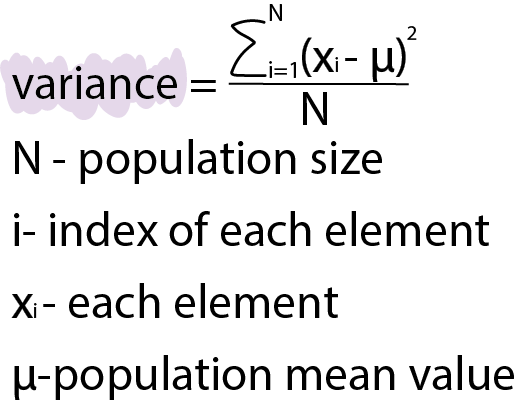Course Content

# Learning Statistics with Python

Learning Statistics with Python

##Population Variance

Variance

Variance measures how far numbers are spread from the mean.

Look at the distribution of salaries from our dataset:

Formula

The formula is different for the sample and population. In this chapter, we will calculate population variance.Explanation

The population variance is the sum of the squares of the result of the subtracting population mean from each value of data divided by the number of elements of the population.

Everything was clear?

Section 3. Chapter 1